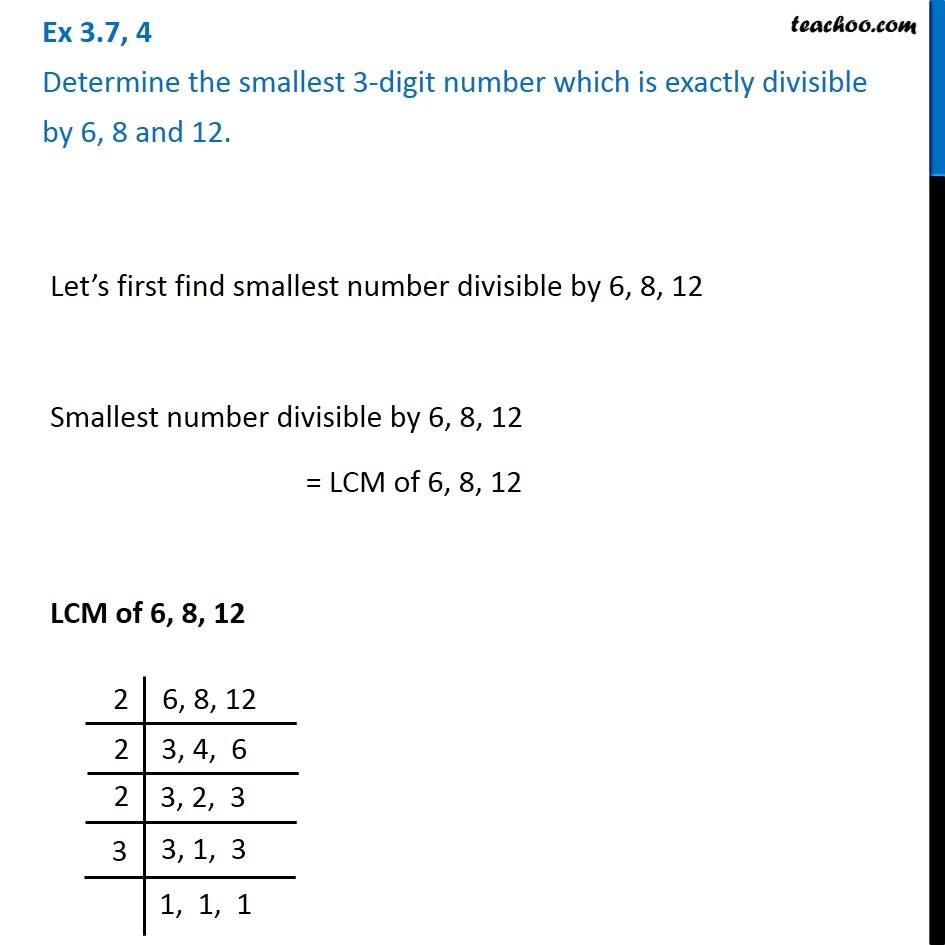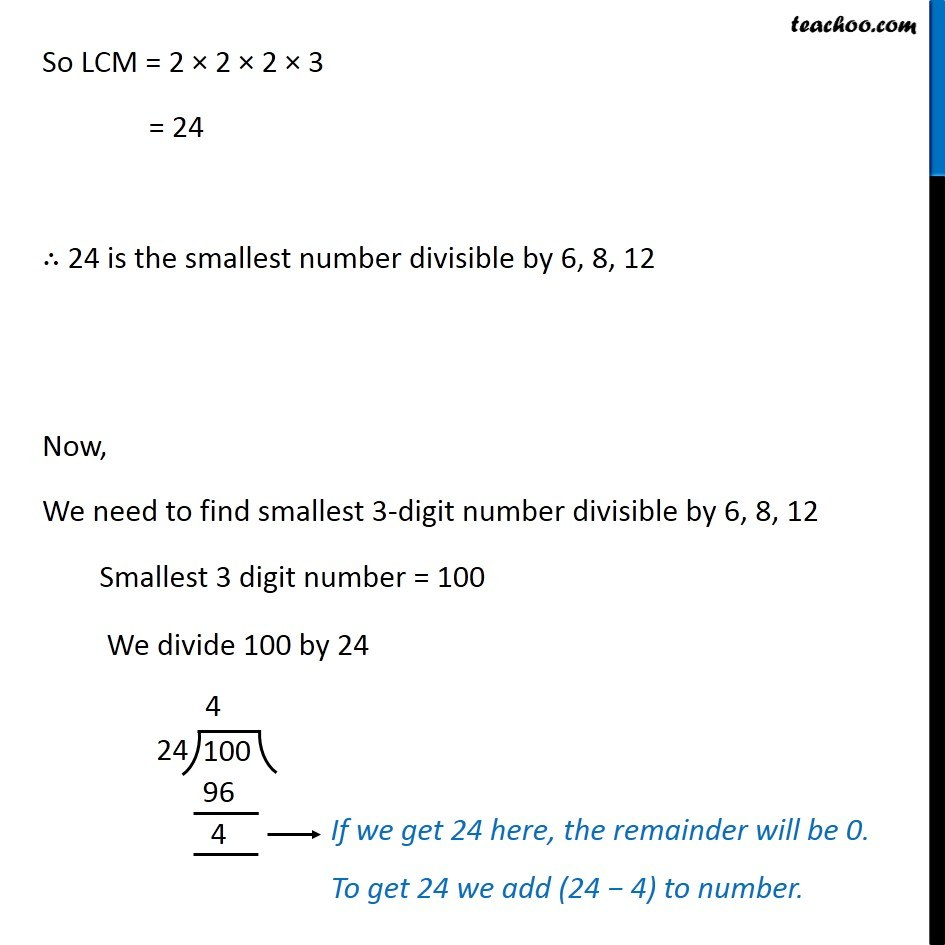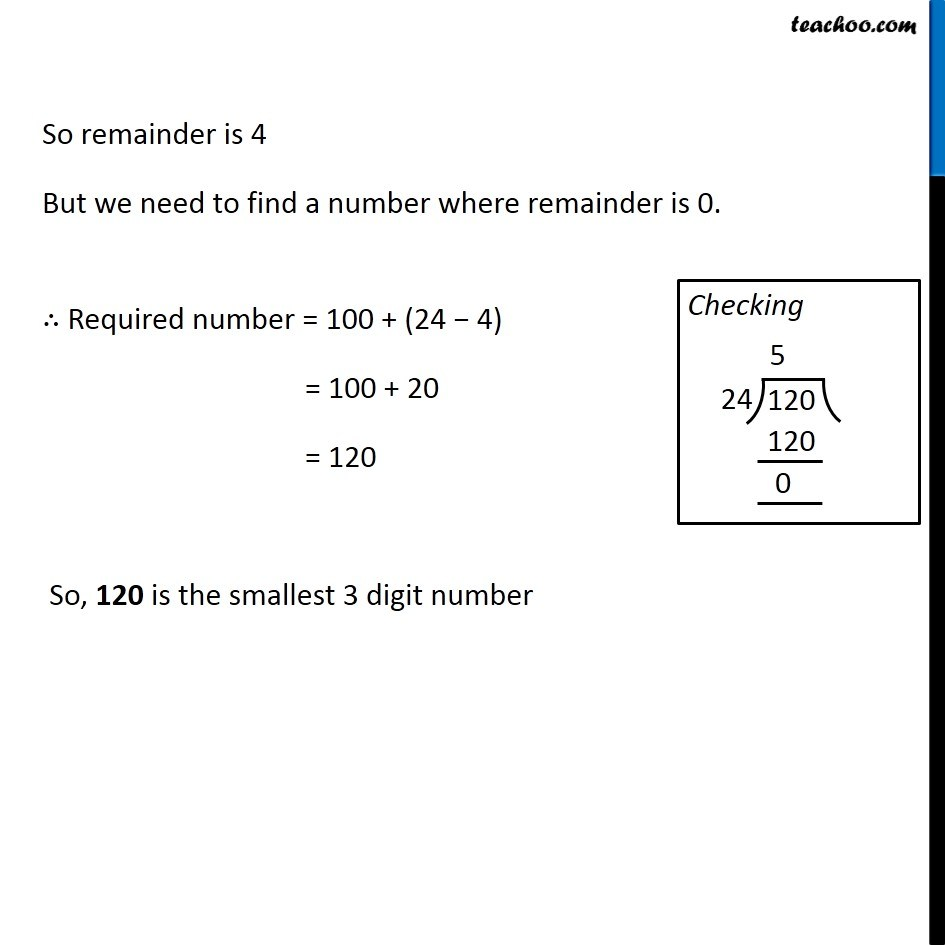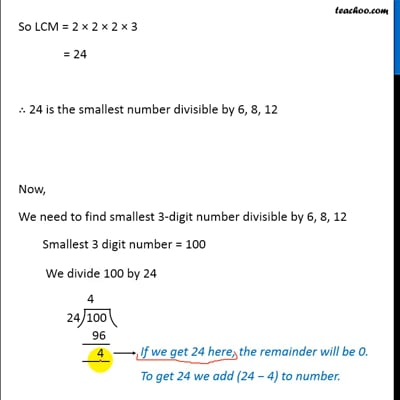Statement questions on LCM

Chapter 3 Class 6 Playing with Numbers
Concept wiseThis video is only available for Teachoo black users

### Transcript

Let’s first find smallest number divisible by 6, 8, 12 Smallest number divisible by 6, 8, 12 = LCM of 6, 8, 12 LCM of 6, 8, 12 So LCM = 2 × 2 × 2 × 3 = 24 ∴ 24 is the smallest number divisible by 6, 8, 12 Now, We need to find smallest 3-digit number divisible by 6, 8, 12 Smallest 3 digit number = 100 We divide 100 by 24 So remainder is 4 But we need to find a number where remainder is 0. ∴ Required number = 100 + (24 − 4) = 100 + 20 = 120 So, 120 is the smallest 3 digit number